Others

# Battery power system

Subjects: Energy & Fuel Technology View times: 437

## Abstract

Ambient (or operating) temperature and cell (surface) temperature affects the state-of-charge (SOC) estimation of battery in electric vehicles and smart grid systems. A unified battery cell model that considered both the ambient and cell temperature, hysteresis voltage dynamics and thermal aging on cell’s capacity is required in battery management system (BMS) design.

An ambient and cell temperature-dependent equivalent circuit model (ECM) in Figure 1 is used.  The ambient  temperature refers to the operating temperature where the battery cell operates while the cell temperature is the surface temperature of the battery cell. The battery cell used was obtained from cylindrical  lithium iron phosphate battery cells (ANR26650M1-B) and  the cell simulation model can be obtained from the website .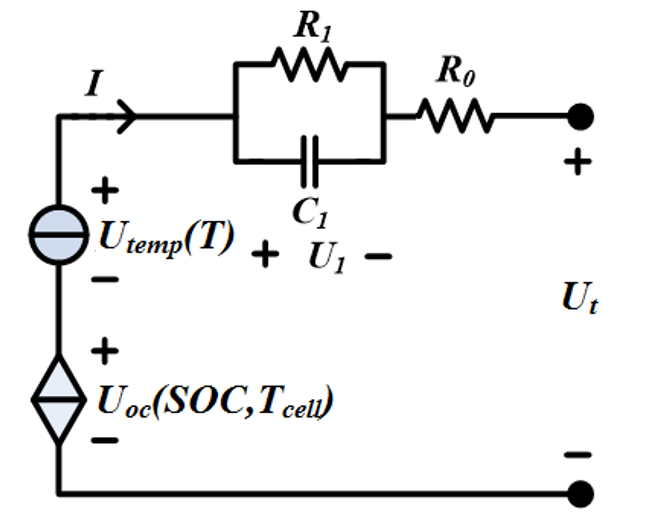Figure 1. Circuit model of temperature-dependent ECM of a battery cell

The terms are incorporated into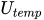to represent the temperature dependency in obtaining the terminal voltage as shown in Figure 2. The plot was taken at ambient temperature of 25oC.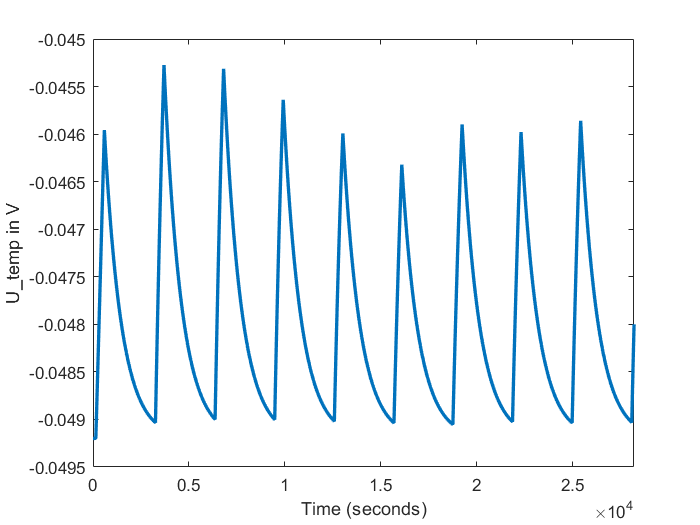Figure 2.as a function of ambient and cell temperature

The resistances and capacitance as a function of ambient temperature and state-of-charge (SOC) can be seen in Figure 3 to 5.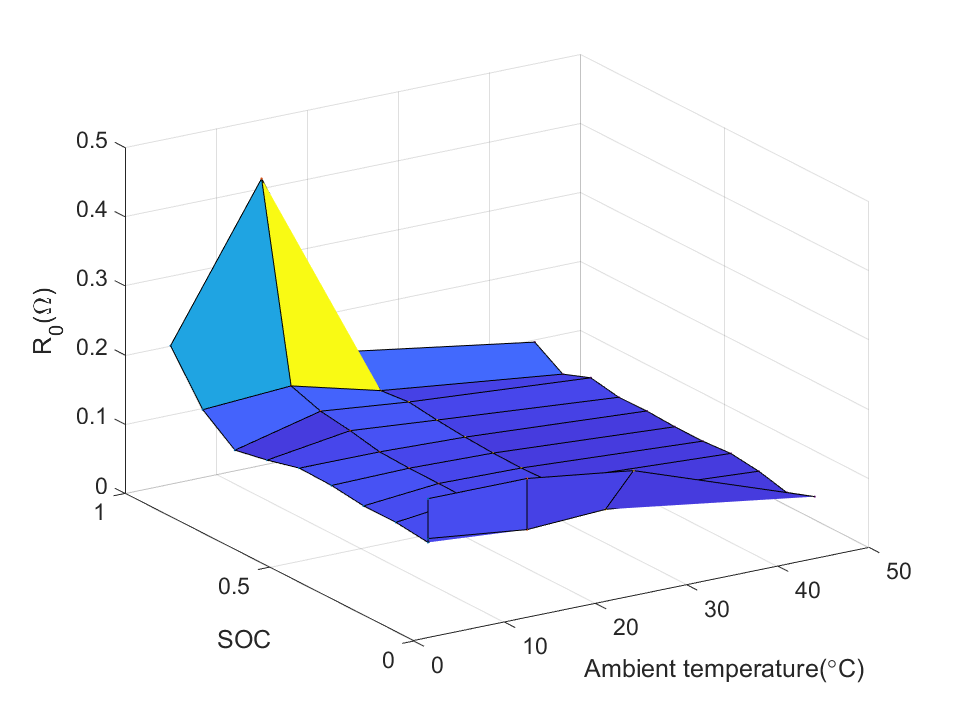Figure 3.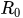values as a function of SOC and ambient temperature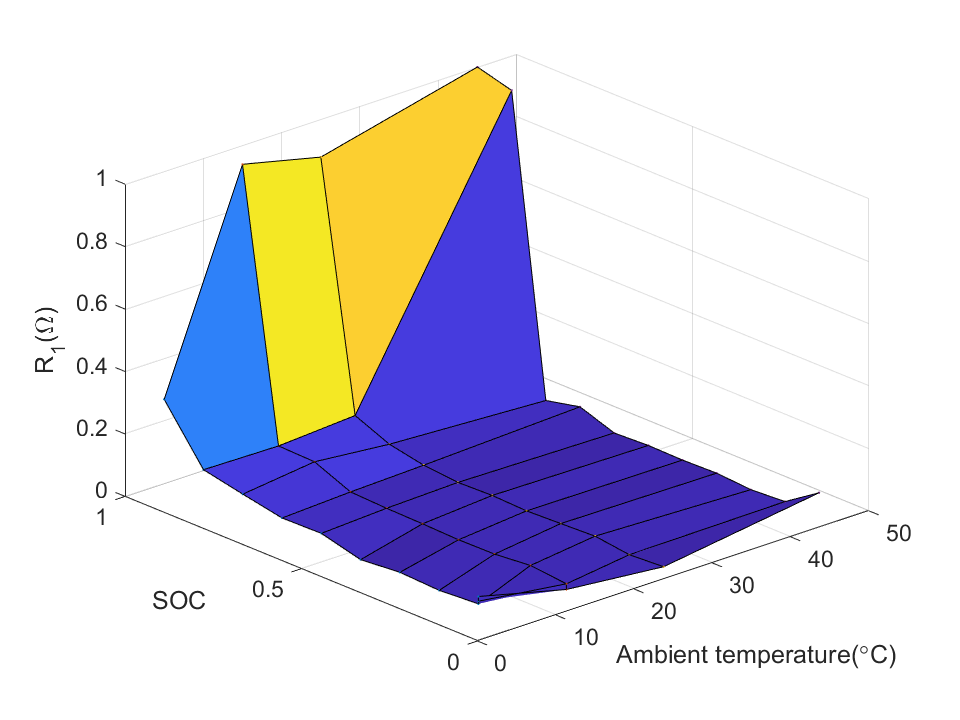Figure 4.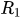values as a function of SOC and ambient temperature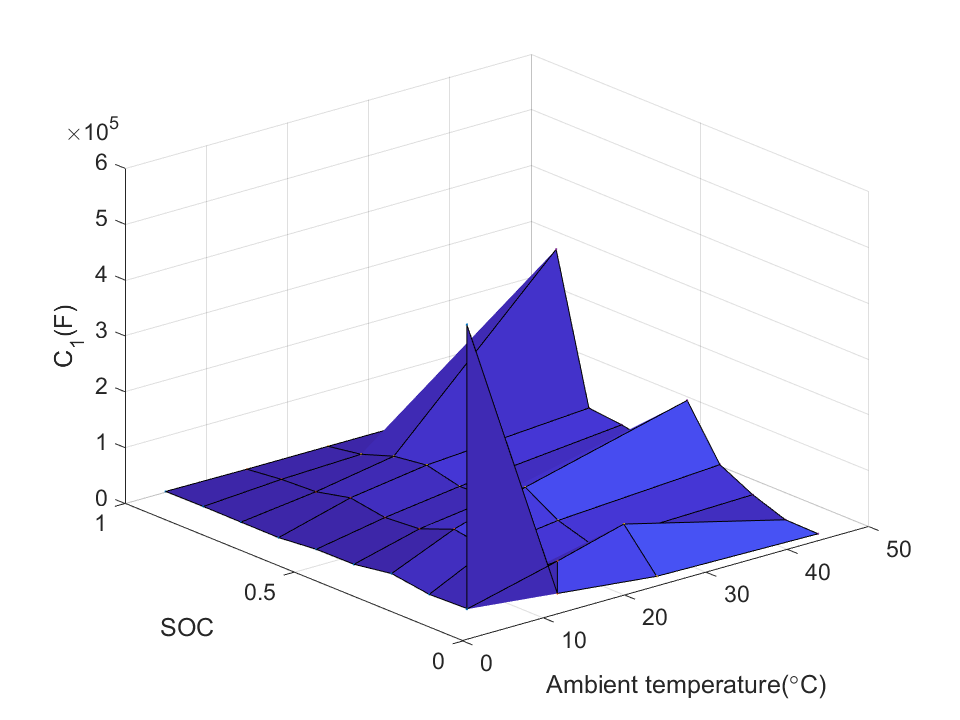Figure 5.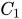values as a function of SOC and ambient temperature

In addition, the open-circuit voltage (OCV) is a function of SOC and ambient temperature as observed in Figure 6.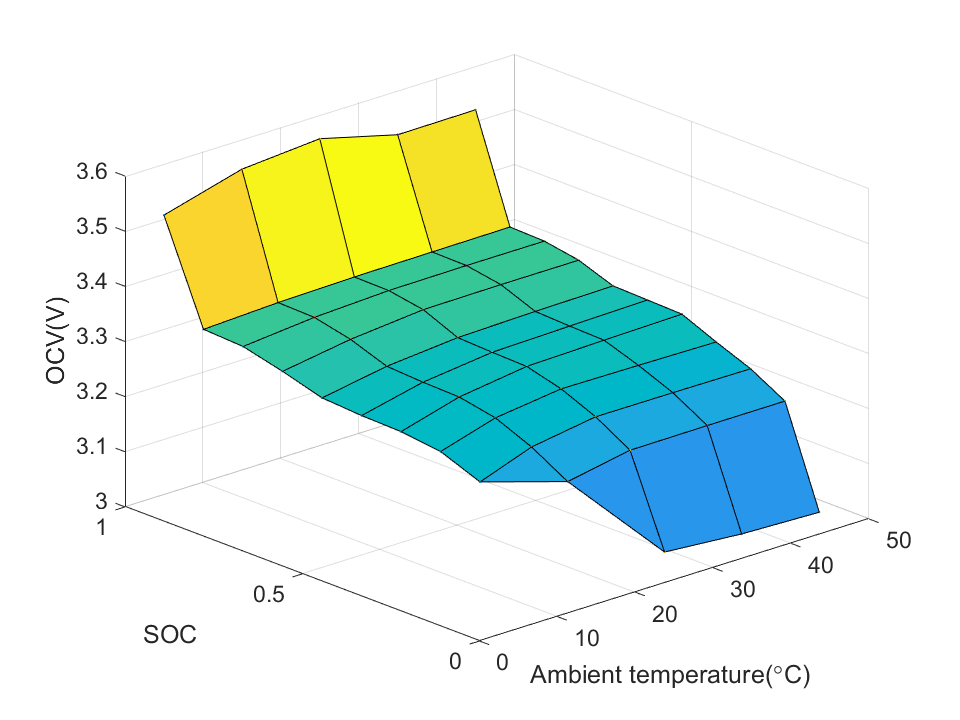Figure 6. OCV values as a function of SOC and ambient temperature

The proposed battery cell model also provides the current-voltage behavior compensated by the hysteresis effect,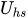for the battery cell model. The curve forcan be seen in Figure 7 where the load current  and SOC profile are plotted below it.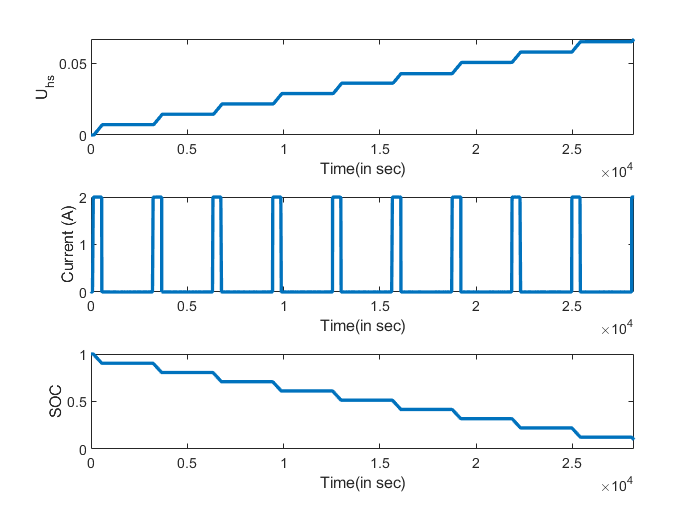Figure 7.as a function of SOC and load current

The time response of is modeled using the model proposed by Bernardi et al. . The plot can be seen in Figure 8 where the ambient temperature of the cell was set at 25oC.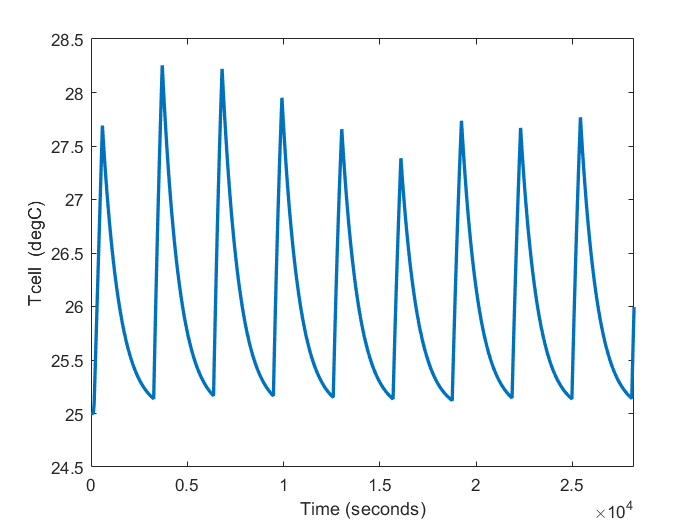Figure 8. Cell temperature at ambient temperature of 25oC

The terminal voltage at ambient temperatures such as 25oC can be seen in Figure 9. It can be observed that the simulated terminal voltage matches experimental data near to the end of discharging cycle or at a low SOC value.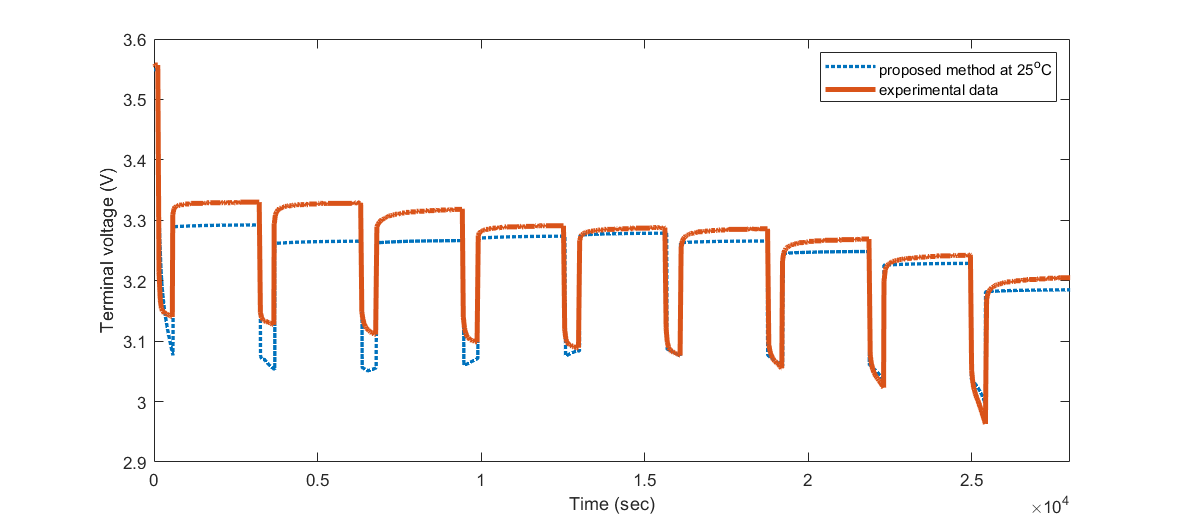Figure 9. Terminal voltage at ambient temperature of 25oC

As seen in Figure 11, with the extended Kalman filter (EKF) on the proposed cell model in (1) to (5), the SOC values converge to the experimental data obtained by Ah counting method. The maximum root mean square error (RMSE) of the resulting SOC estimate is around 0.09.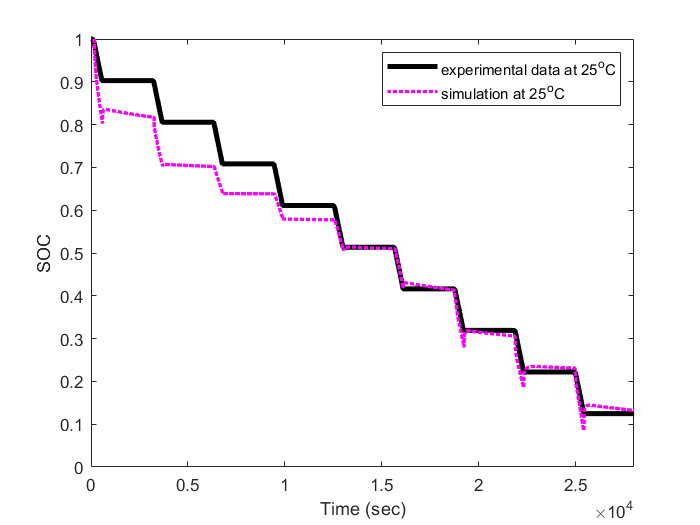Figure 10. SOC  estimate as compared to experimental result (by Ah counting method) obtained at ambient temperature of 25o

## References

1. Z. C. Gao; C. S. Chin; W. D. Toh; J. Chiew; J. Jia; State-of-Charge Estimation and Active Cell Pack Balancing Design of Lithium Battery Power System for Smart Electric Vehicle. Journal of Advanced Transportation 1970, 2017, 1-14, 10.1155/2017/6510747.
2. Zuchang Gao; Cheng Siong Chin; Joel Hay King Chiew; Junbo Jia; Caizhi Zhang; Design and Implementation of a Smart Lithium-Ion Battery System with Real-Time Fault Diagnosis Capability for Electric Vehicles. Energies 1970, 10, 1503, 10.3390/en10101503.
3. Zuchang Gao; Cheng Siong Chin; Wai Lok Woo; Junbo Jia; Integrated Equivalent Circuit and Thermal Model for Simulation of Temperature-Dependent LiFePO4 Battery in Actual Embedded Application. Energies 1970, 10, 85, 10.3390/en10010085.
4. Cheng Chin; Zuchang Gao; State-of-Charge Estimation of Battery Pack under Varying Ambient Temperature Using an Adaptive Sequential Extreme Learning Machine. Energies 1970, 11, 711, 10.3390/en11040711.
5. W.D. Toh; B. Xu; J. Jia; C.S. Chin; J. Chiew; Z. Gao; Lithium Iron Phosphate (LiFePO4) Battery Power System for Deepwater Emergency Operation. Energy Procedia 1970, 143, 348-353, 10.1016/j.egypro.2017.12.695.
6. D. Bernardi; E. Pawlikowski; J. Newman; A General Energy Balance for Battery Systems. Journal of The Electrochemical Society 1970, 132, 5, 10.1149/1.2113792.